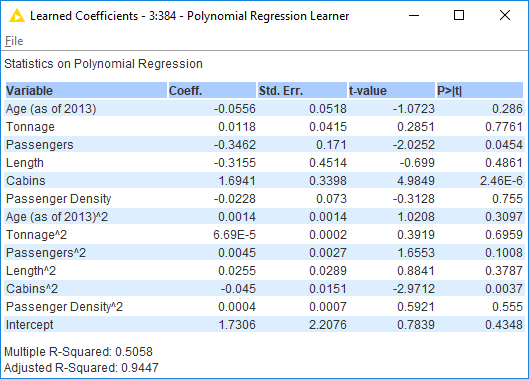# Regression Equations

Hi,

I used gradient boosted trees, random forest, tree ensemble and polynomial regression methods in my model. I want to learn how can i get the prediction regression equations for this models ? Is it possible?

Best Regards,
Gökhan

Hi @gokhan_sir -

You’re not going to get the equations directly, but you can build them based on the information in the coefficients table.So in this case Y = 1.7306 - 0.045*(Cabins^2) - 0.3462*(Passengers) + etc